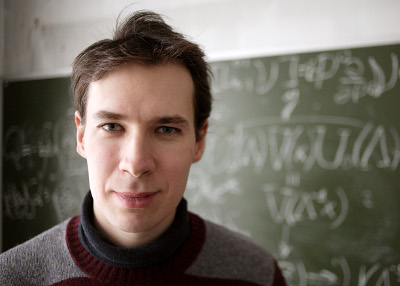In our works we apply quantum field methods developed initially to describe interactions between elementary particles to the problems of statistical physics, namely to the fully developed hydrodynamic turbulence. The general feature of such models of statistical physics like critical behaviour and turbulence is presence of strong fluctuations, and, therefore, the presence of divergences in perturbation theory and absence of small perturbation parameter. In this case the main role is played by ideas of symmetries, remormalization (the system do not depend on the details of physics in small scales), universality (the system depends only on the global characteristics like dimensionality of the space) and scaling.

The theory of critical behaviour states that universal characteristics of the systems under consideration (critical exponents, rations of the amplitudes, scaling functions) depends only on the tensor nature of the field and dimension of the space and can be calculated in the framework of the some systematical expansion. For the turbulent motion the multiscaling behavior is characteristic: the correlation functions of different orders are described by infinite set of different independent exponents. Moreover, the perturbation theory is constructed using the Reynolds number which is not small for the turbulent motion.

During the talk we will discuss how the initial stochastic problem with random noise can be reformulated via some quantum field theory and how renormalization group technique allows us to work with not small perturbation parameter and summarize infinite (asymptotical) series of divergent Feynman diagrams.

by N. Gulitskiy
Department of High Energy Physics and Elementary Particles, Saint Petersburg State University

at SJ1S24 (video conference room) Jesenná 5, PF UPJŠ Košice
on Thursday April 04, 2019
from 14:15